# Differential Transistor Amplifiers

## Discrete Semiconductor Devices and Circuits

• #### Question 1

 Don’t just sit there! Build something!!

Learning to mathematically analyze circuits requires much study and practice. Typically, students practice by working through lots of sample problems and checking their answers against those provided by the textbook or the instructor. While this is good, there is a much better way.

You will learn much more by actually building and analyzing real circuits, letting your test equipment provide the “answers” instead of a book or another person. For successful circuit-building exercises, follow these steps:

1. Carefully measure and record all component values prior to circuit construction, choosing resistor values high enough to make damage to any active components unlikely.
2. Draw the schematic diagram for the circuit to be analyzed.
3. Carefully build this circuit on a breadboard or other convenient medium.
4. Check the accuracy of the circuit’s construction, following each wire to each connection point, and verifying these elements one-by-one on the diagram.
5. Mathematically analyze the circuit, solving for all voltage and current values.
6. Carefully measure all voltages and currents, to verify the accuracy of your analysis.
7. If there are any substantial errors (greater than a few percent), carefully check your circuit’s construction against the diagram, then carefully re-calculate the values and re-measure.

When students are first learning about semiconductor devices, and are most likely to damage them by making improper connections in their circuits, I recommend they experiment with large, high-wattage components (1N4001 rectifying diodes, TO-220 or TO-3 case power transistors, etc.), and using dry-cell battery power sources rather than a benchtop power supply. This decreases the likelihood of component damage.

As usual, avoid very high and very low resistor values, to avoid measurement errors caused by meter “loading” (on the high end) and to avoid transistor burnout (on the low end). I recommend resistors between 1 kΩ and 100 kΩ.

One way you can save time and reduce the possibility of error is to begin with a very simple circuit and incrementally add components to increase its complexity after each analysis, rather than building a whole new circuit for each practice problem. Another time-saving technique is to re-use the same components in a variety of different circuit configurations. This way, you won’t have to measure any component’s value more than once.

• #### Question 2

A qualitative model for transistor behavior is that of a variable resistor. While grossly inaccurate in a quantitative (numbers) sense, it at least provides a means of assessing its behavior in the sense of “more conductive” or “less conductive”.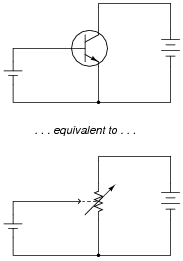Apply this “model” of transistor behavior to this single-transistor amplifier circuit, and describe what happens to the collector voltage (VC) and emitter voltage (VE) when the input voltage (Vin) increases and decreases:• #### Question 3

Identify the type of transistor amplifier this is (common-collector, common-emitter, or common-base), and identify whether it is inverting or noninverting.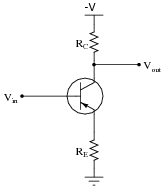Also, explain how to derive the voltage gain equation for this amplifier:

$$A_V= \frac{R_C}{R_E+r'_e}$$

• #### Question 4

Identify the type of transistor amplifier this is (common-collector, common-emitter, or common-base), and identify whether it is inverting or noninverting.Also, explain how to derive the voltage gain equation for this amplifier:

$$A_V= \frac{R_E}{R_E+r'_e}$$

• #### Question 5

Identify the type of transistor amplifier this is (common-collector, common-emitter, or common-base), and identify whether it is inverting or noninverting.Also, explain how to derive the voltage gain equation for this amplifier:

$$A_V= \frac{R_C}{r'_e}$$

• #### Question 6

Would you characterize this transistor amplifier as being inverting or noninverting, with the base terminal of transistor Q2 being considered the input? Explain your answer.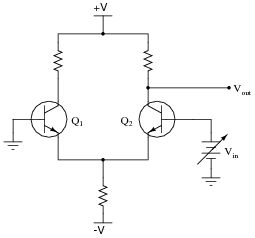• #### Question 7

Would you characterize this transistor amplifier as being inverting or noninverting, with the base terminal of transistor Q1 being considered the input? Explain your answer.• #### Question 8

Here, a differential pair circuit is driven by an input voltage at the base of Q2, while the output is taken at the collector of Q2. Meanwhile, the other input (Q1 base) is connected to ground:Identify what types of amplifier circuits the two transistors are functioning as (common-collector, common-emitter, common-base) when the differential pair is used like this, and write an equation describing the circuit’s voltage gain. Here is another schematic, showing the transistors modeled as controlled current sources, to help you with the equation: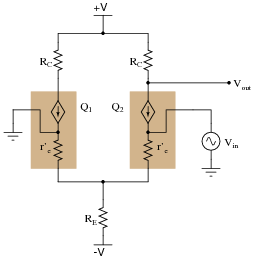• #### Question 9

Here, a differential pair circuit is driven by an input voltage at the base of Q1, while the output is taken at the collector of Q2. Meanwhile, the other input (Q2 base) is connected to ground: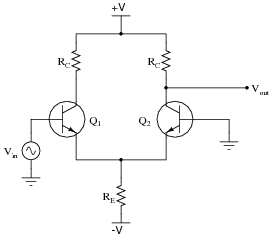Identify what types of amplifier circuits the two transistors are functioning as (common-collector, common-emitter, common-base) when the differential pair is used like this, and write an equation describing the circuit’s voltage gain. Here is another schematic, showing the transistors modeled as controlled current sources, to help you with the equation: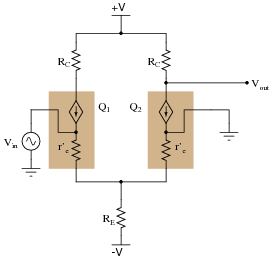• #### Question 10

Write an approximate equation describing the differential voltage gain for a differential pair circuit such as this, in terms of the component values:• #### Question 11

Describe what happens to each of the output voltages (Vout1 and Vout2) as the input voltage (Vin) decreases: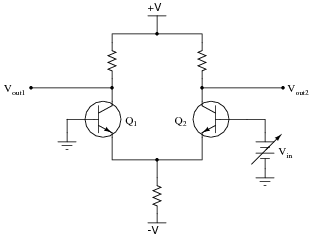• #### Question 12

Suppose this differential-pair circuit was perfectly balanced. In this condition, how much voltage would be expected between the two transistors’ collector terminals?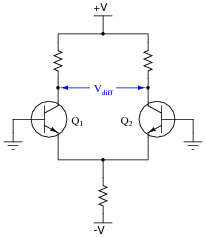What would happen to this differential voltage (Vdiff) if transistor Q2 were to increase in temperature, while transistor Q1 remained at the same temperature? Explain your answer.

• #### Question 13

This circuit is part of a weather monitoring station. Wind speed is measured by the voltage output from a permanent-magnet DC generator, turned by a set of vanes. A light bulb lights up when the wind speed passes a threshold (“trip”) value, established by the potentiometer: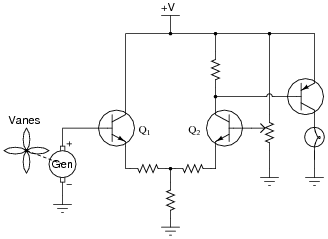Based on your understanding of differential pair circuits, is this a high-speed wind indicating circuit or a low-speed wind indicating circuit?

• #### Question 14

What is common-mode voltage, and how should a differential amplifier (ideally) respond to it?

• #### Question 15

If we connect the two transistor bases together in a differential pair circuit, it can only see common-mode input voltage (no differential input voltage):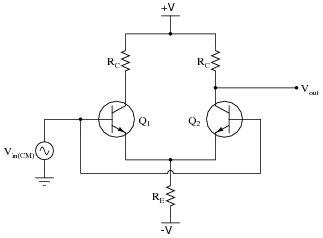An important performance parameter of any differential amplifier is its common-mode voltage gain. Ideally, a differential-input amplifier should ignore any and all common-mode voltage, but in reality there is always some amplification of common-mode voltage. We need to figure out how much of that there will be in any differential-pair circuit.

To help us analyze this circuit (with both inputs tied together so it only sees common-mode input voltage), I will re-draw it in such a way that reflects the symmetrical nature of the circuit: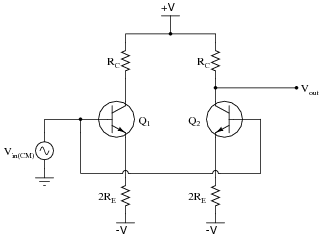First, explain why this re-drawing is justified, and then write the equation describing the common-mode voltage gain of this circuit, in terms of the component values.

• #### Question 16

An improvement to the resistor-based differential amplifier design is the addition of a constant-current source where the two transistors’ emitter currents mesh together:What does the constant-current source “look like” to the rest of the amplifier, in terms of equivalent resistance? What advantage does this give to the amplifier’s performance, over the (simpler) resistor design? Finally, how is this constant-current source actually constructed in a typical differential amplifier circuit?

• #### Question 17

Common-mode rejection ratio is the ratio between a differential amplifier’s differential voltage gain and its common-mode voltage gain:

$$CMRR=\frac{A_{V(diff)}}{A_{V(CM)}}$$

The greater this parameter’s value, the better the differential amplifier will perform as a truly differential amplifier. Combine the equations for differential voltage gain and for common-mode voltage gain for the following differential amplifier circuit, into a single equation for CMRR: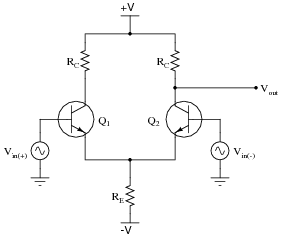• #### Question 18

Differential amplifiers often make use of active loads: a current mirror circuit to establish collector currents between the two transistors, rather than load resistors.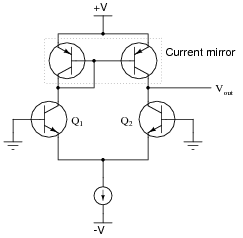What does the current mirror “look like” to the common-emitter side of the differential amplifier circuit, when we apply the Superposition theorem? What aspect of the differential amplifier’s performance is primarily enhanced with the addition of the current mirror to the circuit?

• #### Question 19

Predict how the operation of this differential pair circuit will be affected as a result of the following faults. Consider each fault independently (i.e. one at a time, no multiple faults):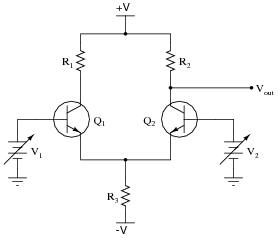Resistor R1 fails open:
Resistor R2 fails open:
Resistor R3 fails open:
Solder bridge (short) across resistor R3:

For each of these conditions, explain why the resulting effects will occur.